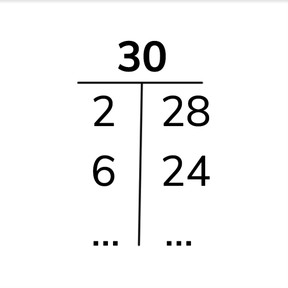Number bond charts to 30

# Number bond charts to 30

Number bond charts to 308,000 schools use Gynzy92,000 teachers use Gynzy1,600,000 students use Gynzy

## General

Students practice decomposing the numbers 21 to 30 by completing number bond charts.

## Relevance

It is useful to decompose numbers to know what is left of a number. If there are 23 people and 12 men, when we decompose the number we discover that there should be 11 women.

## Introduction

Ask students to write down which numbers together compose 20. Check their answers by drawing lines between the correct numbers.

## Development

Explain how you can easily decompose 30 with an example in a restaurant. You have 30 people. Half of the want coffee. How many then want tea? Compose to the ten from the given number and count on to 30. Make the jumps on the number line visible to the students. How many did you count on? This is the number that you put in your decomposition. Show the number bond chart of 30. Show that in the left column the number always goes up by one, and in the right column that the number always goes down by one. Ask if students can com up with other decompositions of 30. Practice in the same way with decomposing 23. Ask students to complete the number bond chart without context (images or support story(.

Check that students understand number bond charts to 30 by asking the following questions:
- How do you complete a number bond chart?
- What are tips for decomposing numbers bigger than 20? (skip counting and counting on)
- Why is it useful to be able to decompose numbers?

## Guided Practice

Students first practice completing number bond charts with half of the numbers (left column) completed. Then they practice decomposing with either column, and finally with non sequential numbers in the number bond charts.

## Closing

Students complete a number bond chart with the numbers out of order. Discuss why it is useful to be able to decompose numbers and remind them that they can use skip counting to help them count on.

## Teaching Tip

Students who have difficulty completing the number bond charts can be supported by representing the number on a number line or using MAB (blocks). Some students will be able to see the decomposition without counting.

## Instruction materials

MAB blocks

### The online teaching platform for interactive whiteboards and displays in schools

• Save time building lessons

• Manage the classroom more efficiently

• Increase student engagement